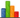Back to Chart version
GDPS (GEM-GLB) forecast for Lat:36.4 Long:33.9
Time Zone: UTC (UTC +0 hrs)

#### Tabular display

- Units are metric

Columns can reordered by dragging the column headers, and sorted by clicking the headers. Use the Add/Remove Columns button to add and remove weather elements. More details about the data can be found below the table.

View Fire Weather version for Prometheus software

Data Dictionary:
• TMP: Temperature, 2 meters above ground, units = Celsius.
• DPT: Dew Point, 2 meters above ground, units = Celsius.
• RH: Relative Humidity, 2 meters above ground, units = Percent.
• WS: Wind Speed, 10 meters above ground, units = km/h.
• WD: Wind Direction, 10 meters above ground, units = degrees true.
• APCP: Accumulated Precipitation, surface, units = millimeters.
• CLOUD: Cloud coverage, total in all levels, units = Percent of sky covered.
• SLP: Sea Level Pressure, units = millibars.
• PTYPE: Precipitation Type (RA = rain, SN = snow, PL = ice pellets, FZRA = freezing rain).
• RQP: Accumulated rain, surface, units = millimeters.
• SQP: Accumulated snow water equivalent, surface, units = millimeters (NOTE: Also equals approximate snowfall in centimeters. ie. 1mm of snow water equivalent = 1cm of fresh snow, on average for a fresh snowfall).
• FQP: Accumulated freezing rain, surface, units = millimeters.
• IQP: Accumulated ice pellets, surface, units = millimeters.
• WS925: Wind Speed, at 925mb (approx 2500ft ASL), units = km/h.
• WD925: Wind Direction, at 925mb, units = degrees true.
• TMP850: Temperature, 850 mb, units = Celsius.
• WS850: Wind Speed, at 850mb (approx 5000ft ASL), units = km/h.
• WD850: Wind Direction, at 850mb, units = degrees true.